Sunday, 30 September 2012

CBSE Class 9 - Science - CH 3 - Atoms and Molecules

Atoms and MoleculesMolecular Structure
NCERT Solution, MCQs, Study Notes, Q & A

Q1: Who established the two important laws of chemical combinations?

Answer: Antoine L. Lavoisier and Joseph L. Proust

Q2: What are the laws of chemical combinations?

Answer: These are group of laws which describe how different elements and compounds combine together to form new compounds. Most of these were established based on chemical experimental results. These laws are:

1. Law of Conservation of Mass
2. Law of Constant composition (or definite proportions)
3. Law of Multiple proportions
4. Law of reciprocal proportions
5. Law of Combining Volumes (Gay Lussac's law of Gaseous Volumes)
[Note: CBSE Class 9 syllabus covers first two laws only. In class 11, you will revisit all of these.]

Q3: What is the law of conservation of mass?

Answer: The law states "During any physical change or chemical change, the total mass of the products remains equal to the total mass of the reactants."
It means during a chemical reaction matter is neither created nor destroyed. It is also called the Law of indestructibility of matter.

Q4: Give an example to show Law of conservation of mass applies to physical change also.

Answer: When ice melts into water, is a physical change. Take a piece of ice in small flask, cork it and weight it (say Wice gm). Heat the flask gently and ice (solid) slowly melts into water (liquid). Weigh the flask again (Wwater gm). It is found there is no change in the weight i.e. Wice= Wwater.
`                              Heat(Δ)`
`                        Ice  ----------➜   Water`
This shows law of conservation of mass holds true for physical changes.

Q5: Explain with example that law of conservation of mass is valid for chemical reactions.

Answer:According to this law, total mass of the products (chemicals produced) is equal to the total mass of the reactants (chemicals take part in reaction). e.g. Carbon combines with Sulphur to form Carbon disulphide. The mass of reactants i.e. carbon and sulphur is same mass of products (carbon disulphide).
`                 Carbon + Sulphur -----➜   Carbon DiSulphide`
`                   C    +   S     -----➜     C2S  `
`                   1g   +  5.34g    =       6.34 g `
`                       LHS          =     RHS`

Q6: Is there any exception to law of conservation of mass?

Answer: Later after atoms were discovered, it was found during nuclear reactions this law does not hold good. In a nuclear reaction, some of the mass gets converted into energy, as given by famous Einstein's mass-energy relationship (E = mC2). The law was rechristened as Law of conservation of mass and energy.

Lavosier and "Reign of Terror"

Remember French Revolution and Robespierre's Reign of Terror. Lavosier was declared as traitor. Within a day, he was tried, convicted and executed. Later French Government admitted her mistake for the false conviction of him.

Lavosier made important contributions in the field of chemistry and biology. He was a pioneer of analytical chemistry. He discovered the gas oxygen and gave its name. He concluded air is mixture of gases and oxygen supports breathing and combustion.

Q7(NCERT): In a reaction, 5.3 g of sodium carbonate reacted with 6 g of ethanoic acid. The products were 2.2 g of carbon dioxide, 0.9 g water and 8.2 g of sodium ethanoate. Show that these observations are in agreement with the law of conservation of mass.
sodium carbonate + ethanoic acid  → sodium ethanoate + carbon dioxide + water

sodium carbonate + ethanoic acid  → sodium ethanoate + carbon dioxide + water

Mass of  sodium carbonate (Na2CO3) = 5.3 g
Mass of ethanoic acid (CH3COOH) = 6g
Mass of carbon dioxide (CO2) = 2.2g
Mass of Water (H2O) = 0.9 g
Mass of sodium ethanoate (CH3COONa) = 8.2 g

Total mass of reactants = 5.3g + 6g = 11.3g
Total mass of products = 2.2g + 0.9 g + 8.2g = 11.3g

Since, Total mass of reactants = Total mass of products, the observations in this reaction are in agreement with the law of conservation of mass.

Q8(CBSE 2011): 2.8 g of nitrogen gas was allowed to react with 0.6 g of hydrogen gas to produce 3.4 g of ammonia. Show that these observations are in agreement with the law of Conservation of mass.

mass of nitrogen (N2) gas (reactant) = 2.8g
mass of hydrogen (H2) gas (reactant) = 0.6g
mass of ammonia gas (NH3) (product) = 3.4 g

The reaction is
nitrogen + hydrogen  → ammonia

Total mass of reactants = 2.8g + 0.6g = 3.4g
Total mass of product = 3.4g

Since Total mass of reactants = Total mass of products, the observations in this reaction are in agreement with the law of conservation of mass.

Q9(CBSE 2011): If 12 g of carbon is burnt in the presence of 32 g of oxygen, how much carbon dioxide will be formed?

carbon + oxygen → carbon dioxide
Mass of carbon = 12g
Mass of oxygen = 32g
Mass of carbon dioxide (CO2) = xgJ L Proust
Since the reaction is in agreement with law of conservation of mass, then
Total mass of reactants = Total mass of products
i.e. 12g + 32g = x
i.e. x = 44g
∴ mass of carbon dioxide (CO2) formed = 44g

Q10: Who proposed Law of Definite Proportions (or Law of Constant Composition)?

Answer: J.L. Proust 1799 (French Chemist)

Q11: State Law of constant proportions. Explain with an example.
OR
Q(CBSE 2011): (i) State the law of constant proportions.
(ii) Show that water illustrates the law of constant proportions.

Answer: In a chemical substance the elements are always present in definite proportions by
mass, no matter how that chemical compound is prepared.

Example: Water always contains Hydrogen and Oxygen in the same proportion  i.e. the ratio of
the mass of hydrogen to the mass of oxygen is always 1:8, whatever the source of water. Whether water comes from melting of ice or by condensation of steam (physical changes) or by it is produced through chemical reactions.

Q12(NCERT): Hydrogen and oxygen combine in the ratio of 1:8 by mass to form water. What mass of oxygen gas would be required to react completely with 3 g of hydrogen gas?

Answer: Given that the ratio of hydrogen and oxygen by mass to form water is 1 : 8.
⇒ the mass of oxygen gas required to react completely with 1 g of hydrogen gas =  8 g of O2
∴ 3g of hydrogen (H2) gas requires = 3 × 8 = 24g of O2

Q13(NCERT): A 0.24 g sample of compound of oxygen and boron was found by analysis to contain 0.096 g of boron and 0.144 g of oxygen. Calculate the percentage composition of the compound by weight.

Answer: Given, mass of boron = 0.096 g
mass of the oxygen = 0.144g
mass of the sample compound (boron+oxygen) = 0.24 g
According to Law of definite proportions, boron and oxygen are in fixed ratio in the given compound.= 0.096:0.0144  = 2:3

⇒ % of boron = mass of boron × 100 / mass of compound = 0.096 × 100/0.24 = 40%and % of oxygen = mass oxygen  × 100 / mass of compound = 0.144 × 100/0.24 = 60%

Q14(NCERT): When 3.0 g of carbon is burnt in 8.00 g oxygen, 11.00 g of carbon dioxide is produced. What mass of carbon dioxide will be formed when 3.00 g of carbon is burnt in 50.00 g of oxygen? Which law of chemical combination will govern your answer?

`                Carbon + Oxygen ➜ Carbon Dioxide`
`                  3g   +  8g    =   11g `

⇒ Total mass of reactants = Total mass of products
∴ Law of conservation of mass is obeyed.

It also shows that carbon dioxide (CO2) contains carbon and oxygen in fixed ratio i.e. 3:8 which follows Law of Constant proportion.

⇒ 3g of carbon (C) will react with 8g of oxygen (O) to give 11g of carbon dioxide (CO2).
⇒ Remaining oxygen (50 -8 = 42g) will not participate in the reaction.

Q14(CBSE 2011): Magnesium and oxygen combine in the ratio of 3 : 2 by mass to form magnesium oxide. How much oxygen is required to react completely with 12 g of magnesium?

Answer: Magnesium (Mg) and Oxygen (O) combine in the ratio = 3:2 to form compound magnesium oxide (MgO). It follows Law of Constant proportions.
⇒ 3g of Mg combines with = 2g of O.
∴ 12g of Mg combines with = 2 × 12/3 = 8g of O.

Q15: What are the postulates of Dalton's atomic theory?

Answer: Dalton's theory states that matter, whether an element, a compound or a mixture is composed of small particles called atoms.
The  postulates are:
1. All matter is made of very tiny particles called atoms.
2. Atoms are indivisible particles i.e. can neither be created or destroyed in a chemical reaction. (Law of Conservation of mass)
3. Atoms of a give element have same mass and identical chemical properties.
4. Atoms of different elements have different masses and chemical properties.
5. Atoms combine in the ratio of small whole numbers to form compounds. A chemical reaction is a re-arrangement of atoms. (Law of Multiple Proportions)
6. The relative number and kinds of atoms are constant in a given compound. (Law of Definite Proportions)
Q16(NCERT): Which postulate of Dalton’s atomic theory is the result of the law of conservation of mass?

Answer: The postulate of Dalton’s atomic theory i.e. "Atoms are indivisible particles, which can neither be created nor destroyed in a chemical reaction" is the result of law of conservation of mass.

Q17(NCERT): Which postulate of Dalton’s atomic theory can explain the law of definite proportions?

Answer: The postulate of Dalton's atomic theory that explains the law of definite proportions is:
The relative number and kinds of atoms are constant in a given compound.Boltzman
Suicide of Ludwig Boltzman (1844-1906)

When Dalton proposed his theory (1804), it was not well accepted by many scientists, because there was no experiment to prove atoms exist. However few scientists believed in his theory. Ludwig Boltzman, famous Austrian physicist, was firm the believer in atoms and used this concept to explain kinetic theory of gases. Unfortunately it did not go well with other scientists (Ostwald and Mach) who criticized it badly. This had a grave impact on Boltzman. His health deteriorated and eventually he committed suicide. Within couple of years after his death, scientists confirmed experimentally and convinced the world that atoms do exist. Later, J.J. Thomson was the first one to propose a model for the structure of an atom.

Q18(MCQ): Which of the following statements is NOT true about an atom?
(a) Atoms are the building blocks from which molecules and ions are formed.
(b) Atoms cannot exist independently.
(c) Atoms are neutral in nature
(d) Atoms combine together to form matter that we can see, feel or touch.

Answer: (b) Atoms cannot exist independently.

Q19:  What is an atom?

Answer: An atom is the smallest particle of an element which takes part in chemical reactions. An atom is considered to be building blocks of matter.STM Image of Gold Atoms credits:wikicommons
Q20: Why is it not possible to see an atom with naked eyes?

Answer: The size of an atom is so small that it cannot be seen by naked eye or by simple optical instruments. e.g. Considering an atom a sphere, the radius of Hydrogen atom is approximately 10nano-metres (1 nm = 10-9m).

To see atoms of different elements, a special type of microscope called scanning tunnelling microscope  (STM) is used.

Nano-science and Nano-technology:
With the invention of scanning tunneling microscope, a new branch of science and engineering emerged called Nano-science and Nano-technology. It deals in designing functional systems at atomic and molecular scale i.e. of size in nano-meters. At nano-scale, laws of Newton fail. It follows principles of Quantum Physics.

Q20: Who proposed the chemical notation based on first two letters of the name of the element?

Q21: Name the international organization who approves names of elements.

Answer: IUPAC (International Union of Pure and Applied Chemistry)

Q22: What is the chemical symbol for iron?

Q23: Name five elements have single letter chemical symbol.

Answer: Hydrogen (H), Oxygen(O), Boron(B), Sulphur(S), Carbon(C) and Nitrogen (N)

Q24: Name the element having following Latin names
(i)   Stibium
(ii)  Cuprum
(iii) Argentum
(iv) Natrium
(v)  Stannum
(vi) Wolfram
(vii) plumbum
(viii) Kalium

(i)   Stibium - Antimony (Sb)
(ii)  Cuprum - Copper (Cu)
(iii) Argentum - Silver(Ag)
(iv) Natrium - Sodium (Na)
(v)  Stannum - Tin (Sn)
(vi) Wolfram - Tungeston (W)
(viii) Kalium - Potassium (K)

Q25: Write the chemical symbols of  the following:
(i) Gold
(ii) Iron
(iii) Chlorine
(iv) Mercury

(i) Gold - Au
(ii) Iron - Fe
(iii) Chlorine - Cl
(iv) Mercury - Hg

Q26: How will you define chemical symbol?

Answer: A chemical symbol of an element is a shorthand chemical notation for the full name of an element.

Q27: What is the significance of a chemical symbol?

Answer: The symbol of an element has the following significance:
1. symbol stands for the name of the element in while representing chemical formula and chemical equations.
2. it stands for one atom of the element.
3. it represents quantity of the element in terms of mass, moles or gram-atomic mass etc. while computing chemical reactions.
Q28: Can atoms of an element exist independently? Give examples of elements which exist in atomic form. Give examples of elements that do not exist in atomic form.Water Molecule

Answer:  Atoms of most elements do not to exist independently. There are few elements like Helium (He), Argon (Ar), Krypton(Kr), Radon(Rn), Neon(Ne) and Xenon(Xe) - collectively called noble gases, exist at atomic form. Atoms of other elements combine together and form molecules and
ions. These molecules or ions aggregate in large numbers to form the matter.  e.g.
• Hydrogen (H2), Oxygen(O2), Nitrogen(N2) etc. atoms of the same element combine together and form molecules.
• Water (H2O), Carbon Dioxide(CO2), Common Salt(NaCl) etc. atoms of different elements combine together and form molecules.
• Na+, Cl-, Cu2+ etc. atoms of elements form ions in aqueous solutions.
Q29: Why do atoms of the most of the elements not exist independently?

Answer: Atoms of the most of the elements are reactive by nature. They are not able to exist independently and form molecules and ions.

Q30: Which element has the smallest atom in size?

Q31(NCERT): What is the atomic mass unit?

Answer: The mass equal to 1/12th of the mass of  126C atom is called the one atomic mass unit. It is 1.66 × 10-27 kg.  It is represented by symbol 'u' (older symbol 'amu').

1 u  = 1/12th of the mass of carbon-12 atom.

Q32: Magnesium is two times heavier than C-12 atom, what shall be the mass of Mg atom in terms of atomic mass units? (Given mass of C-12 atom = 12u)

Answer:  1 u = 1/12th of C-12 atom
mass of C-12 atom = 12u
mass of Mg is twice of C-12 atom = 2 ×12u  = 24u

Q33(CBSE 2011): What is relative atomic mass  of an element? How it is related to atomic mass unit?

Answer: The relative atomic mass (Ar) of an element is the average relative mass of an element of the element with respect to an atom of Carbon-12 taken as 12u.

`                                      Average mass of 1 atom of an element `
`Relative Atomic mass of an element = -------------------------------------- `
`                                      1/12th of Mass of one C-12 atom`

The relative atomic mass is a pure number and has no units. It indicates number of times one atom of an element is heavier than 1/12th of C-12 atom.

Relative atomic mass is also called average atomic mass
Atomic mass is expressed in amu or u while relative atomic mass is a number.

e.g. Atomic mass of one Hydrogen atom is 1u and its relative atomic mass is 1.

Q34: Define molecule. What are its important properties?

Answer: The smallest particle of matter (element or compound) which can exist in a free state is called a molecule.

Atoms of the same element or of different elements can join together to form molecules.

Properties:
1. Molecules of any given substance are similar.
2. Molecules of different substances are different.
3. The properties of a substance are the properties of the molecule of that substance.
4. Each molecule is described by its chemical formula.Buckminsterfullerene consists of carbon atoms only (credits:wikipedia)
Q34: Based on type of substance, how molecules are classified?

1. elementary molecules: molecules formed by atoms of the same element. e.g. Chlorine (Cl2), Hydrogen (H2), Oxygen(O2), Nitrogen(N2) gases, Buckminsterfullerene (C60) etc.
2. compound molecules: molecules formed by atoms of different elements e.g. (H2O), Carbon Dioxide(CO2), Glucouse (C6H12O6), Aspirin (C9H8O4)Aspirin Molecule

Q35: What is atomicity?

Answer: The number of atoms contained in a molecule of a substance (element or compound) is called its atomicity.

Q36: Based on atomicity, how molecules are categorized?

1. Monoatomic molecules (1 atom molecule)
2. Diatomic molecules (2 atoms molecule)
3. Triatomic molecules (3 atoms molecule)
4. Tetraatomic molecules (4 atoms molecule)
5. Polyatomic molecules (more than 4 atoms molecule)
Q37: Give three examples of monoatomic molecules.

Answer: Noble gases. e.g. Helium (He), Neon(Ne) and Argon(Ar).

Q38: Give four examples of diatomic molecules.

Answer:  Chlorine (Cl2), Hydrogen (H2),Carbon Monoxide (CO), Sodium Chloride (NaCl)

Q39: (i) What is the chemical formula of Water molecule?
(ii) What is its atomicity?
(iii) Calculate the ratio of masses of atoms of elements present in water molecule.
(iv) Calculate the ratio by number of atoms of elements present in water molecule.

(i) Water molecule:  (H2O)
(ii) It is triatomic i.e. 2 atoms of H + 1 atom of O = 3 atoms of water molecule.
(iii) Mass of 1 H-atom = 1 u
Mass of two H-atoms = 2u
Mass of O atom = 16u
Mass ratio of H:O = 2u:16u = 1:8
(iv) Number of atoms ration H:O = 2:1

Q40: What is an ion?

Answer: An ion is positively or negatively charged atom or group of atoms. There are two types of ions:
1. cations (positively charged ions) e.g.Na+, K+, Cu2+
2. anions (negatively charge ions) e.g. Cl-, SO42-

Q41(NCERT): What are polyatomic ions? Give examples?

Answer: A group of atoms carrying a charge is known as a polyatomic ion.
Examples are: Sulphate anion (SO42-), carbonate anion (CO32-), Ammonium cation (NH41+) etc.

Q42: Give examples of triatomic molecules.

Answer: Ozone (O3), Carbon Dioxide(CO2), Nitrogen Dioxide (NO2)

Q43: What is valency of an element?

Answer: The valency of an element is defined as its capacity to combine with other elements. It can be be used to find out how the atoms of an element will combine with the atom(s) of another element to form a chemical compound.

Elements may have valency: 1(monovalent), 2 (divalent), 3(trivalent) and 4 (tetravalent) respectively. Some elements show variable valency in different compounds.
e.g. In red oxide of Copper called Copper (I) Oxide (Cu2O), Cu has valency=1. While in black oxide of Copper i.e. Copper(II) Oxide (CuO), Cu is divalent (valency=2).

Q44(NCERT): What is meant by the term chemical formula?

Answer:  A chemical formula is a representation of chemical compound using a set of chemical symbols that forms the compound. It may be an empirical formula or a molecular formula. The molecular formula represents the actual number of atoms of elements present in the compound. While empirical formula tells the whole number ratio of atoms of elements present in the compound.

e.g. The empirical formula of Water is HO which tells it consists of hydrogen and oxygen atoms. The chemical (molecular) formula of Water is : H2O, which tells 2 Hydrogen atoms combine with one atom of oxygen.
The chemical formula of a molecular compound is determined by the valency of each element.

Q45(NCERT): Write down the formulae of
(i) sodium oxide
(ii) aluminium chloride
(iii) sodium suphide
(iv) magnesium hydroxide

(i) sodium oxide: Na+ + O2- ➜Na2O
(ii) aluminium chloride: Al3+ + Cl- ➜ AlCl3
(iii) sodium suphide: Na+ + S2- Na2S
(iv) magnesium hydroxide: Mg2+ + OH- Mg(OH)2

Q46(NCERT): Write down the names of compounds represented by the following formulae:

(i) Al2(SO4)3
(ii) CaCl2
(iii) K2SO4
(iv) KNO3
(v) CaCO3

(i) Al2(SO4)3: Aluminium Sulphate
(ii) CaCl2: Calcium Chloride
(iii) K2SO4:Potassium Sulphate
(iv) KNO3: Potassium Nitrate
(v) CaCO3 : Calcium Carbonate

Q47(NCERT): Write the chemical formulae of the following. Also identify the ions present.
(a) Magnesium chloride
(b) Calcium oxide
(c) Copper nitrate
(d) Aluminium chloride
(e) Calcium carbonate.

(a) Magnesium chloride: MgCl2 (ions present are: Mg2+, Cl1- )
(b) Calcium oxide: CaO (ions present are: Ca2+, O2- )
(c) Copper nitrate: Cu(NO3)2 (ions present are: Cu2+, NO31- )
(d) Aluminium chloride: AlCl3 (ions present are: Al3+, Cl1- )
(e) Calcium carbonate: CaCO3 (ions present are: Ca2+, CO32- )

Q48(NCERT): Give the names of the elements present in the following compounds.
(a) Quick lime
(b) Hydrogen bromide
(c) Baking powder
(d) Potassium sulphate.

(a) Quick lime: Calcium Oxide (CaO), Elements Present: Calcium(Ca), Oxygen(O)
(b) Hydrogen bromide: HBr, Elements Present: Hydrogen (H), Bromine(Br)
(c) Baking powder: Sodium Bicarbonate (NaHCO3) Elements Present: Sodium(Na), Hydrogen(H), Carbon(C) and Oxygen(O).
(d) Potassium sulphate: K2SO4Elements Present:Potassium(K), Sulphur(S), Oxygen(O)

Q49(NCERT): How many atoms are present in a
(i) H
2S molecule and
(ii) PO43- ion?

(i) H2S molecule: Three atoms (2 H-atoms + 1 S atom)
(ii) PO43- ion: Five atoms (1 P-atom + 4 O-atoms)

Q50(CBSE 2011): (a) Write a chemical formula of a compound using zinc ion and phosphate ion.
(b) Calculate the ratio by mass of atoms present in a molecule of carbon dioxide.
(Given C =12, O =16)

Answer: (a) Zinc ion: Zn2+, Phosphate ion: PO43-
⇒ Zinc Phosphate: Zn3(PO4)2

(b) Carbon DiOxide (CO2) = 1 C-atom + 2 O-atoms
Atomic mass of 1 C-atom = 12u
Atomic mass of 1 O atom = 16u
Atomic mass of 2 O-atoms = 32u
Ratio by mass of C:O atoms = 12u:32u = 3:8

Q51: What is the molecular mass of a substance?

Answer: The average mass of a molecule of a substance expressed in atomic mass units is called its molecular mass.
It is obtained by adding together the atomic masses of all the atoms present in one molecule of the substance.

Q52: What is Formula Unit Mass? How it is different from molecular mass?

Answer:   The formula unit mass of a substance is a sum of the atomic masses of all atoms in a
formula unit of a compound. Formula unit mass is calculated in the same manner as we calculate the molecular mass.
The difference is that we use the word formula unit for ionic compounds.

Q53(NCERT): Calculate the molecular masses of H2, O2, Cl2, CO2, CH4, C2H6, C2H4, NH3, CH3OH.

(i) molecular mass of H2 = 2 × Atomic mass of H = 2 × 1u = 2u
(ii) molecular mass of O2 = 2 × Atomic mass of O = 2 × 16u = 32u
(iii) molecular mass of Cl2 = 2 × Atomic mass of Cl = 2 × 35.5u = 71u
(iv) molecular mass of  CO2 = 1 × Atomic mass of C + 2 × Atomic mass of O
= 1 × 12u + 2 × 16u = 12u + 32u = 44u
(v) molecular mass of CH4= 1 × Atomic mass of C + 4 × Atomic mass of H
= 1 × 12u + 4 ×1u = 12u + 4u = 16u
(vi) molecular mass of C2H6 =  2 × Atomic mass of C + 6 × Atomic mass of H
=  2 × 12u + 6 ×1u = 24u + 6u = 30u
(vii) molecular mass of C2H4 =  2 × Atomic mass of C + 4 × Atomic mass of H
=  2 × 12u + 4 ×1u = 24u + 4u = 28u
(viii) molecular mass of NH3 = 1 × Atomic mass of N + 3 × Atomic mass of H
= 1  × 14u + 3 × 1u = 14u + 3u = 17u
(ix) molecular mass of CH3OH =  1 × Atomic mass of C + 4 × Atomic mass of H + 1 × Atomic mass of O = 1 × 12u + 4 × 1u + 1 × 16u = 12u + 4u + 16u = 32u

Q54(NCERT): Calculate the formula unit masses of ZnO, Na2O, K2CO3, given atomic masses of Zn = 65 u, Na = 23 u, K = 39 u, C = 12 u, and O = 16 u.

(i) Formula unit mass of  ZnO = 1 × Atomic mass of Zn + 1 × Atomic mass of O = 1 × 65u + 1 ×16 u
= 65u + 16u = 81u
(ii) Formula unit mass of  Na2O =  2 × Atomic mass of Na + 1 × Atomic mass of O
= 2 × 23u + 1 × 16u = 46u + 16u = 62u
(iii) Formula unit mass of  K2CO3= 2 × Atomic mass of K + 1 × Atomic mass of C + 3 × Atomic mass of O
= 2 × 39u + 1 × 12u + 3 × 16u = 78u + 12u + 48u = 138u

Q55: What are ionic compounds?

Answer: Compounds which are made up of ions (cation + anion) are called ionic compound. In ionic compounds (e.g. NaCl),  the positively charged ions (cations, e.g. Na1+) and negatively charged ions (anions, e.g. Cl1-) combine together due to strong forces of attraction between them.

Q56(CBSE 2011): How do we know the presence of atoms if they do not exist independently for most of the elements?

Answer: Matter consists of tiniest particles which cannot be further divided. Elements combine together to form compounds and Law of Combinations prove that elements combine in definite proportions to form a particular compound. It implies atoms of various elements combine together and form molecules. Each molecule can be described by a unique chemical formula.

Q57(CBSE 2011): An element 'Z' forms the following compound when it reacts with hydrogen, chlorine, oxygen and phosphorus.
ZH3, ZCl3, Z2O3 and ZP
(a) What is the valency of element Z?
(b) Element 'Z' is metal or non-metal?

Answer: The valency of element Z is 3. It is a metal, it combines with non-metals like Hydrogen(H), Chlorine(Cl), Oxygen and Phosphorous(P).

Q58(CBSE 2011): Name one element each which forms diatomic and tetra atomic molecule.

Answer: Diatomic: Oxygen, Tetra Atomic: Phosphorus

Q59: Name one element which forms diatomic and triatomic molecule.

Answer: Oxygen (forms diatomic oxygen O2 gas and triatomic ozone O3 gas molecules).

Q60: What is gram-atomic mass of an element?

Answer: When atomic mass of an element is expressed in grams, it is called the gram-atomic mass of the element.
e.g. Atomic mass of Oxygen (O) = 16u
Gram-atomic mass of oxygen = 16g

Q61: What is gram-molecular mass of a substance?

Answer: The molecular mass of substance expressed in grams is called the gram-molecular mass of the substance.

e.g. molecular mass of Hydrogen (H2)molecule = 2u
Gram-molecular mass of Hydrogen (H2)molecule = 2g

Q62: Define mole. What is its significance?

Answer: A mole (or mol) is the amount of a substance which contains the same number of chemical units (atoms, molecules or ions) as there are atoms in exactly 12 grams of pure carbon-12.

Since 12g of  carbon-12 is found to contain 6.023 × 1023 atoms of C-12. Therefore, a mole represents a collection of 6.023 × 1023 chemical units (atoms, molecules or ions). This number 6.023 × 1023 is also called Avagadro's Number (NA or NO).

Significance of mole: A mole represents the following
1. It represents quantity in number i.e. 6.023 × 1023 chemical units.
2. The mass of 1 mole  of an element is equal to 6.023 × 1023 atoms of the element.
3. 1 mol of a substance represents one gram-formula mass of the substance i.e.
1 mole of atoms of an element = Gram atomic mass of the element.
1 mole of molecules of a substance = Gram-molecular mass of the substance.
e.g. 1 mole of Oxygen (O2) molecules = 6.023 × 1023 molecules = 32g of O2
4. One mole of gas at standard temperature and pressure occupies 22.4 litres volume (Avagadro's Law).

Q63(CBSE 2011): What is molar mass?

Answer: Mass of 1 mole of any substance is called its molar mass (M). It is measured as grams/mol  (g/mol) or kg/mol. For an element, its molar mass is equal to its gram atomic mass. For compounds (or molecules), its molar mass is equal to its gram-molecular mass.
e.g. Gram-molecular mass of  Hydrogen (H2)  = 2g
⇒ Molar mass of  Hydrogen (H2) = 2g/mol.

Q64: Who introduced the term 'mole' in chemistry?

Q65: When 'mole' was chosen internationally standard way to express larger number of chemical units?

Q66: How many moles are there in 4.6 gms of Sodium(Na)?

Answer: Number of moles = mass in gm ÷ atomic mass
Atomic mass of Na = 23.
moles = 4.6g/23 = 2/10 = 0.2 moles

Q67(NCERT): If one mole of carbon atoms weighs 12 gram, what is the mass (in gram) of 1 atom of carbon?

Answer:  1 mol of Carbon atoms = 12g
1 mol of atoms = No atoms = 6.023 × 1023 atoms⇒ 6.023 × 1023 carbon atoms = 12g
∴ mass of 1 carbon atom = 12g ÷ No = 12g / 6.023 × 1023 = 1.992 × 10-23 gram

Q68(NCERT): Which has more number of atoms, 100 grams of sodium or 100 grams of iron (given, atomic mass of Na = 23 u, Fe = 56 u)?

Answer: We know that, 1 mol of atoms of an element has mass = gram atomic mass.
∴ mass of 1 mol of Na atoms = 23g
Or 23g of Na has = 1 mol of atoms
∴ 100g of Na = 100/23 = 4.35 moles

Similarly, 56g of Fe has = 1 mol of atoms
∴ 100g of Fe has = 100/56 = 2.17 moles
⇒ 100g of Na has more number of atoms than 100g of Fe.

Q69(NCERT): What is the mass of
(a) 1 mole of nitrogen atoms?
(b) 4 moles of aluminium atoms (Atomic mass of aluminium = 27)?
(c) 10 moles of sodium sulphite (Na2SO3)?

Answer: (a) 1 mol of nitrogen (N) atoms = gram atomic mass of N = 14g

(b) mass = molar mass × number of moles
1 mol of Aluminium (Al) = gram atomic mass of Al = 27g
mass of 4 mols of Al = 4 × 27 = 108g

(c) mass = molar mass × number of moles
molecular mass of sodium sulphite (Na2SO3) =
=  2 × Atomic mass of Na + 1 × Atomic mass of S  + 3 × Atomic mass of O
= 2 × 23u + 1 × 32u + 3 × 16u = 46u + 32u + 48u
= 126u
mass of 1 mol of sodium sulphite (Na2SO3) = gram molecular mass or molar mass = 126g
mass of 10 moles of Na2SO3 = 10 × 126g = 1260g

Q70(NCERT): Convert into moles:
(a) 12 g of oxygen gas
(b) 20 g of water
(c) 22 g of carbon dioxide
(a) 32g of Oxygen = 1 mol
12g of Oxygen = 12/32 = 0.375 mol
(b) 20g of water
Molar mass of Water (H2O) = 2 × 1 + 16 = 18g
18g of H2O = 1 mol
20g of  H2O = 20/18 = 1.1 mol

(c) Molar mass of  carbon dioxide(CO2) = 12g + 2 × 16g = 12g + 32g = 44g
44g of CO2 = 1 mol
22g of CO2 = 22/44 = 0.5 mol

Q71(NCERT): What is the mass of:
(a) 0.2 mole of oxygen atoms?
(b) 0.5 mole of water molecules?

(a)  mass = molar mass × number of moles
molar mass of Oxygen atoms = 16g
mass of 0.2 mol of Oxygen atoms = 16 × 0.2 = 3.2g

(b) molar mass of water molecule (H2O) = 2 × 1 + 16 = 18g
mass of 0.5 mole of Water = 18 × 0.5 = 9g

Q72: Find out number of atoms in 15 moles of He.

Answer: 1 mole of He has = 6.023 × 1023 atoms
15 moles of He have15 × 6.023 × 1023 = 9.034 × 1024 atoms

Q73(NCERT): Calculate the number of molecules of sulphur (S8) present in 16 g of solid sulphur.

Answer: Gram Atomic mass of Sulphur = 32g
Molar mass of sulphur (S8) = 8 × 32g = 256g
∴ 256g of sulphur (S8) = 6.023 × 1023 molecules
16g of  sulphur (S8) = 6.023 × 1023 × 16 / 256 = 3.76 × 1022 molecules

Q74(NCERT): Calculate the number of aluminium ions present in 0.051 g of aluminium oxide.
(Hint: The mass of an ion is the same as that of an atom of the same element. Atomic mass of Al = 27 u)

Answer: Molar mass of aluminium oxide (Al2O3) = 2 × 27 + 3 × 16 = 102g
⇒ 102g of  aluminium oxide (Al2O3) contains = 1 mole of  molecules = 6.023 × 1023 molecules
The molecular formula of  aluminium oxide = Al2O3
⇒ 1 molecule of aluminium oxide = 2 atoms of aluminum
∴ 102g of  aluminium oxide (Al2O3) contains = 2 × 6.023 × 1023 atoms of Al
∴ 0.051g of aluminium oxide (Al2O3)will have = 2 × 6.023 × 1023× 0.051 / 102
=  6.023 × 1020 atoms of Al

Q75: Calculate the mass percentage of Carbon(C) , Hydrogen (H) and Oxygen (O) in one molecule of glucouse (C6H12O6).
(Atomic mass of C = 12u, H = 1u and O = 16u)

Answer:  Molecular mass of glucouse (C6H12O6) = 6 × 12u + 12 × 1u + 6 × 16u
= 72u + 12u + 86u = 180u
⇒ Molar mass of  glucouse (C6H12O6) = 180 g/mol

% of C = Total mass of C/ Molar mass of glucouse = 6 × 12g × 100/ 180g = 7200/180 = 40%
% of H = Total mass of H/ Molar mass of glucouse =12 × 1g × 100/ 180g = 1200/180 = 6.67%
% of O = Total mass of O/ Molar mass of glucouse =6 × 16g × 100/ 180g = 9600/180 = 53.33%

Q76: Calculate the number of molecules of phosphorus (P4) present in 31 gram of phosphorus.
Answer:  Atomic mass of phosphorus = 31 u
Molecular mass of P4 = 31× 4 = 124 u
⇒ Molar mass of P4 = 124 g/mol.

⇒ Mass of 1 mole ( 6.023 × 1023 ) P4 molecules = 124 g

∴ 124 g of  P4 =  6.023 × 1023 molecules of P4

1g of  P4 = ( 6.023 × 1023 )/(124) = 0.25 × 1023 molecules
31g  P4 =  0.25 × 1023 × 31 = 1.5 × 1023 molecules                           .... answer1.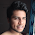Thats what i want to see...
THANKS A LOT MAN.... :-)

1.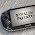Anytime, credits to Eduvictors. :)

2.3.Its gud man thanks a lot.

4.good one can be very helfull

1.that's why it is called good

2.Quiet nitish. You made no sense.

3.seriously nitish u made no sense

5.This comment has been removed by the author.

1.2.if someone doesn't like it pls atleast don't publish unnecessary comments

6.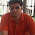Excellent work, Great Keep it up...It will be helpful to many students or aspirants appearing for competition exams.

7.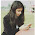Very good :D

8.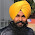i like this very much

9.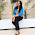10.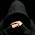its usefull man thank a lottttt..................

11.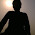it would be useful for me.thanx

12.i did all and enjoyed alot many good ques are there it made me revise the ch vry good...... thnx

13.it was really helpful for me....
cud do well in exams...

14.a little bit useful

15.very simple couldn't you make it difficult

1.simple can be understood better
read yur textbooks you will find it difficult
they give it simple for better understanding

2.Yeah...simple is easy.

16.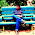Excellent!!
Best thing to revise before Exams (Theory part)

17.Wow. Nice, nigga :D

18.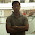19.20.helped me a lot...post some more of them!! :p

21.What a note!!
It helped me a lot...very helpful..

22.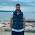Great work keep it up

23.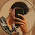good word dude

24.good word dude

25.very good and nice work this helped me a lot in sa-2

26.when 10g of sulphur is burnt in 10g of oxygen 20g of sulphur dioxide is produced ? find the mass of sulphur dioxide formed on burning 20g of sulphur in 30g of oxygen? give answer fast

27.its amazing
helps learning and understanding of concepts easier
and gives worsheets to practise also
i'm speechless

28.Good
29.30.